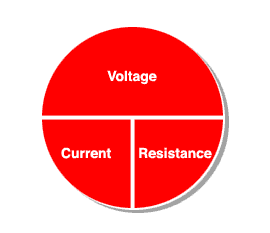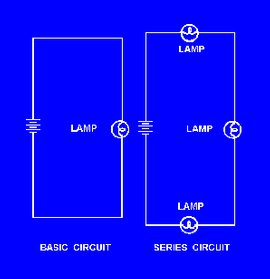Skip to main contentAuto Math Home PageMeasurementDisplacementOhm's LawOhm's Law PracticeAuto CollisionParts, Tools and Tires* Mr. Azria* Mr. Dodge* Mr. McGivney* Mr. Orioli
Ohm's Law Reference Page
 A) Ohm's Law CircleOhm's Law:                            V = A x R   Ohm's law is a mathematical relationship that exists   among circuit voltage (V), current (A), and resistance (R). Terms:   V - Voltage is measured in "volts"   A - Current is measured in "amps"   R - resistance is measured in "ohms" _____________________________________________ Water Analogy:   V is the "pressure" or "push" behind the circuit.   A is the "flow" of electrons through the circuit.   R is the "opposition" to the flow of electrons.

 B. Series CircuitsSeries Circuit Rules: 1. Current has ONE path to flow. 2. Rt = R1 + R2 + R3 +....    (Resistance add up.) 3. Circuit current is CONSTANT throughout the circuit. 4.  There are voltage DROPS over each resistor. 5. If the current path is BROKEN, all current flow stops. (one light goes out, they all go out).

C. Parallel Circuit________________________________

Parallel Circuit Rules

1. Has 2 or more paths for current  to flow.

2. The current total is equal to the sum of each current path.
At = A1 = A2 + A3 +......

3. Voltage is CONSTANT over each load in the circuit.

4. Rt is 1 divided by the sum of the reciprocal of each resistor.
Rt =
1
1/R1 + 1/R2 + 1/R3....

5. If one current path is broken, the current flows into the remaining paths. (if one light goes out, the others stay lit).
 LEARN MORE WITH OCM SCHOOL DISTRICT & EDUCATOR SERVICES QUICKLINKS ABOUTChristopher J. Todd Interim District Superintendent PO Box 4754 Syracuse, NY 13221 (315) 433-2600 www.ocmboces.org

Developed by CNYRIC